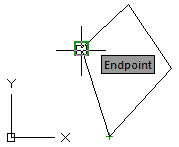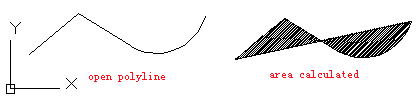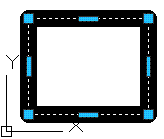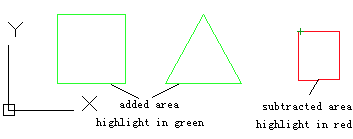# AREA command

## The AREA command is used to calculate the area and circumference of specified object or defined region.

Command

AREA

Ribbon: Utilities > Measure > Area
Menu: Tools > Inquiry > Area

Command Prompts

Specify first corner point or [Object/Add area/Subtract area] <object>:

Function Description:

Users could observe area information by the AREA and MASSPROP commands; as well they could create closed polyline or region by BOUNDARY command and use the LIST command or PROPERTIES palettes to display area.

Relative Glossary:

• Corner point : Calculate area and circumference of region defined by specified points. All points must be in the plane that parallel to XY plane of current UCS.
It will display the virtual line between specified points; all of them form a polygon. Press ENTER to display its circumference definition. If the polygon is open, when calculating its area, it will assume a line from the endpoint to the start point and calculate the surrounded area; when calculating its circumference, the assumed line will be involved.• Object : Calculate area and circumference of selected object. Users could calculate area for circle, ellipse, spline, polyline, polygon, region, and 3D solid.

Note:
• It will not report area of 2D solid (created by SOLID command).
• If the selected polyline is open, there will be an assumed line connecting the last point and the start point. The surrounding area will be calculated. When calculating its circumference, the last assumed line will be ignored.
•• When calculating area and circumference of wide line, its center line will be used.• Add area : In the “Add” mode, it could calculate the total area of defined region. Users could use this option to calculate separate area and circumference of each selected object and calculate the total area of them all.

In this mode, the virtual line will be highlighted in green. Pressing ENTER, it will display area and circumference of al surrounded area and return back to “ADD” mode.

If the polygon is open, when calculating its area, it will assume a line from the endpoint to the start point and calculate the surrounded area; when calculating its circumference, the assumed line will be involved.

• Subtract area : The “Subtract area” is similar to the “Add area” option. Users could use this command to subtract area from specified total area. Under the command prompt, it will display subtracted total area and circumference in the command line. In this mode, the virtual line will be highlighted in red.